Organic (2017 VCE) 1) Which of the following contains a chiral carbon?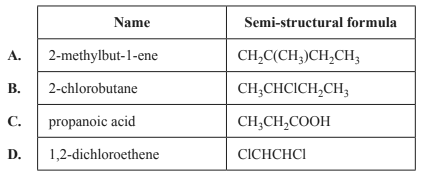Solution2) Which one of the following structures represents a zwitterion of a 2-amino acid?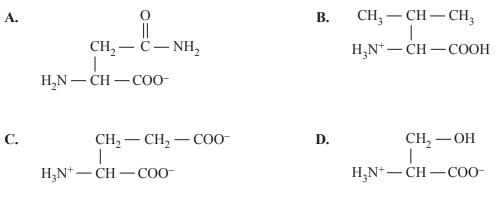Solution3) The glycaemic index (GI) indicates how quickly carbohydrates in food are broken down and raise a person’s blood glucose level. The table below shows the percentage of amylose and amylopectin in the carbohydrate content of four foods, P, Q, R and S. Based on this information, which one of the foods listed would be expected to have the lowest GI value?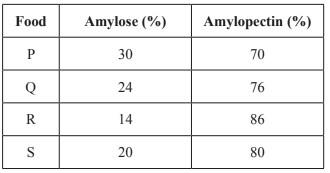Solution4) Industrially, ethanol, C2H5OH, is made by either of two methods. One method uses ethene, C2H4, which is derived from crude oil. The other method uses a sugar, such as sucrose, C12H22O11, and yeast, in aqueous solution. The production of C2H5OH from C12H22O11 and yeast proceeds according to the equation C12H22O11(aq) + H2O(l) → 4C2H5OH(aq) + 4CO2(g) a. Determine the mass, in grams, of pure C2H5OH that would be produced from 1.250 kg of C12H22O11 dissolved in water. M(C12H22O11) = 342 g mol–1 Solutionb. Complete the reaction by i. writing the formula for the reactant "X" below and ii. classify this type of reaction iii. give an appropriate reagent for the final step.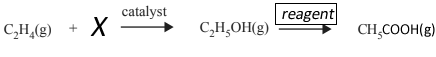Solutionc. CH3COOH can be used in the production of esters. i. Write a balanced chemical equation for the reaction of CH3COOH with propan-1-ol using semi-structural formulas for all organic compounds. ii. Write the IUPAC name for the ester product of the equation above. Solution5) There are a number of structural isomers for the molecular formula C3H6O. Three of these are propanal, propanone and prop-2-en-1-ol. The skeletal structure for the aldehyde propanal is as shown below.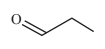i. Write the semi-structural formula for the ketone isomer propanone ii. Draw the structural formula for the isomer prop-2-en-1-ol in the space provided below Solution